# Sparse Matrix Data Structure

## Sparse Matrix Data Structure:

In this article, I am going to discuss Sparse Matrix Data Structure using C and C++ Language with Examples. Please read our previous article, where we discussed Menu Driven Program for Matrices in C and C++ Language with Examples.

##### What is a matrix?

A matrix can be defined as a two-dimensional array having ‘m’ rows and ‘n’ columns. A matrix with m rows and n columns is called an m × n matrix. It is a set of numbers that are arranged in the horizontal or vertical lines of entries.

##### What is Sparse Matrix?

Sparse matrices are those matrices that have the majority of their elements equal to zero. In other words, the sparse matrix can be defined as the matrix that has a greater number of zero elements than the non-zero elements. Now, the question arises: we can also use the simple matrix to store the elements, then why is the sparse matrix required?

##### Why is a sparse matrix required if we can use the simple matrix to store elements?

There are the following benefits of using the sparse matrix –

1. Storage – We know that a sparse matrix contains lesser non-zero elements than zero, so less memory can be used to store elements. It evaluates only the non-zero elements.
2. Computing Time: In the case of searching in the sparse matrix, we need to traverse only the non-zero elements rather than traversing all the sparse matrix elements. It saves computing time by logically designing a data structure traversing non-zero elements.
##### Representation of Sparse Matrix

A sparse matrix is a matrix in which there is more number of zero elements.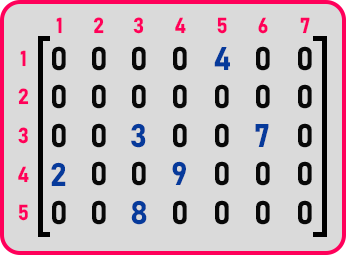Here, we have an example matrix of order 7 by 5 and you can see that it is having very few non-zero elements i.e. 4, 3, 7, 2, 9, 8. There are only 8 non-zero elements and the rest are zeroes. Mostly in the statistical data or survey data, Data may be presented in the form of a matrix and there may be a lot of zero values. If so then if you are using sparse matrices in a program then we may be having a lot of zero values. For which we may be wasting space and also processing time.

So, we want to store a matrix in less amount of space and the processing is also less. So, what we should do? We should store only non-zero elements. For storing non-zero elements, there is more than one approach available.1st method is a coordinate list and the 2nd is a compressed sparse row. The coordinate list method is also called a 3-column representation. So first we will see the coordinate list or 3 column representation method.

##### Coordinate List / 3-Col representation:

In this method, for every non-zero element, we need three things:

1. Row number
2. Column number
3. Element

Let us take the same matrix which we showed above,1st element is present on row no. ‘1’, column no. ‘5’ and element is ‘4’. 2nd element is present on row no. ‘1’, column no. ‘5’ and element is ‘4’. So, we will have a couple of three values that is column number, row number, and the element. So, we can have the list of couples so we can call them coordinates or we can represent them in the form of columns. So that is 3 columns: 1st column is ‘row number’, 2nd column is ‘column number’ and the 3rd column is ‘element’.Now let us represent those non-zero values in these three columns. Element ‘4’ will be filled as: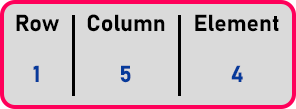Here we have left the 1st-row blank. We will fill this in at the end. Now ‘3’ will be filled as: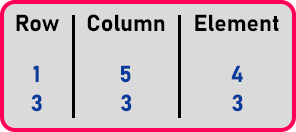Now in this way, we will fill all the elements as: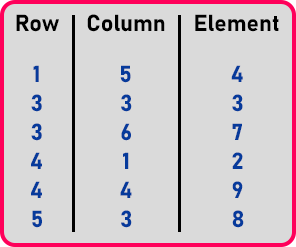Now we will fill the first row as we left it empty. We will use the first row to store the information about the sparse matrix means its dimensions and the total number of non-zero elements. So,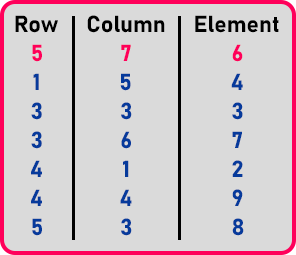Now let us look at the second method which is compressed sparse rows.

##### Compressed Sparse Rows:In this method, a sparse matrix is represented using 3 arrays. 1st array is a set of non-zero elements:We have taken all these non-zero elements in the same order in which they are appearing. Don’t change the order. We need an array for rows. 2nd array will contain the no. of non-zero elements which will present in a row (corresponding array index number) in the sparse matrix:Here, the 0th element of this array will be 0 as indices start in the array from 0, and in matrices, it starts from 1. Next, IA  = ‘1’ as 1st row contains only one element.

• IA  = ‘1’ as the 2nd row doesn’t contain any non-zero element so it will remain the same as previous one.
• IA  = ‘3’, 3rd row contains 2 elements and the previous value is ‘1’ in the array so add these ‘1+2 = 3’.
• IA  = ‘5’, 4th row contains 2 elements and the previous value is ‘3’ in the array so add these ‘3+2 = 5’.
• IA  = ‘6’, 5th row contains only one element so add ‘1’ to the previous value as ‘5 + 1 = 6’.

These values are not showing the number of non-zero elements in a particular row. Suppose we want to know no. of elements in row 5 then,

= A  – A  = 6 – 5 = 1 element.

For row 3,

= A  – A  = 3 – 1 = 2 elements.

In this way, we will fill this array. 3rd array will contain the column number of non-zero elements. Below is the array which contains non-zero elements.Now in the same order, we will column the number of corresponding elements in an array:So as many elements are there that many columns will be there. So, this is another method that is called compressed sparse rows. Let us analyze a little bit upon space.

The dimension of the above matrix is 5×7, which means 35 elements including zero and non-zero elements. And if each element is an integer and is taking 2 bytes so this will be 35 * 2 = 70 bytes. And in a compressed sparse row, how much space is required?

Size of 1st array + 2nd array + 3rd array,

= (6 + 6 + 6) * 2 = 36 bytes. It requires only 36 bytes.

In the next article, I am going to discuss the Addition of Sparse Matrices with Examples. Here, in this article, I try to give a brief Introduction to Sparse Matrix in Data Structure and I hope you enjoy this Sparse Matrix in Data Structure using C and C++ Language article.